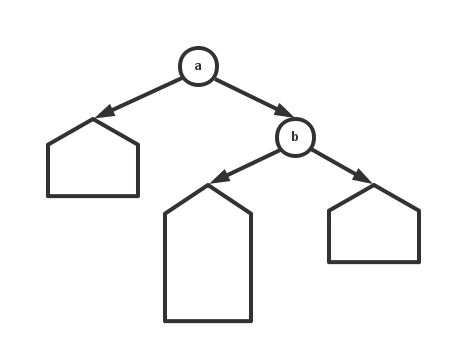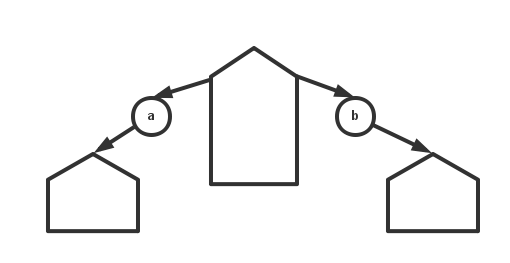# 秩平衡树(Rank Balanced Tree)

riteme: “这是并查集和Treap的杂交品种。”
ruanxz: “ZY！”

## 1 秩

### 1.1 树的秩高

$$nil.\text{rank} = 0 \tag{1.1}$$

$$x.\text{rank} = \max\{x.\text{left}.\text{rank}, x.\text{right}.\text{rank}\} + 1 \tag{1.2}$$

 1 2 function UPDATE(h): h.rank = max(h.left.rank, h.right.rank) + 1## 2 旋转

  1 2 3 4 5 6 7 8 9 10 11 12 13 14 15 16 17 18 19 20 21 22 23 function LEFT-ROTATE(h): ASSERT h.left != nil x = h.left h.left = x.right x.right = h UPDATE(h) UPDATE(x) return x // 使用左子树来替代原来的节点 function RIGHT-ROTATE(h): ASSERT h.right != nil x = h.right h.right = x.left x.left = h UPDATE(h) UPDATE(x) return x // 使用右子树来替代原来的节点 

 1 x = LEFT-ROTATE(x) 

## 3 普通秩平衡树

P: 并查集 x Treap
-> F1: “贼式二叉树”

### 3.1 查询

  1 2 3 4 5 6 7 8 9 10 function QUERY(h, key): if h = nil: return nil // 没有查找到 if key < h.key: return QUERY(h.left, key) // 结果在左子树中 else if key > h.key: return QUERY(h.right, key) // 结果在右子树中 else: return h // 命中节点 

### 3.2 平衡

 1 TOLERANCE = 1 

  1 2 3 4 5 6 7 8 9 10 function BALANCE(h): if h.left.rank > h.right.rank and h.left.rank - h.right.rank > TOLERANCE: // 左子树的秩过大 h = LEFT-ROTATE(h) else if h.left.rank < h.right.rank and h.right.rank - h.left.rank > TOLERANCE: // 右子树的秩过大 h = RIGHT-ROTATE(h) UPDATE(h) // 更新节点 return h // 返回调整后的节点1 2 3 4 5 6 7 8 9 10 11 12 13 14 15 16 17 function BALANCE(h): if h.left.rank > h.right.rank and h.left.rank - h.right.rank > TOLERANCE: // 左子树的秩较大 if h.left.right.rank > h.left.left.rank: // 如果左子树的右儿子的秩较大 h.left = RIGHT-ROTATE(h.left) h = LEFT-ROTATE(h) else if h.left.rank < h.right.rank and h.right.rank - h.left.rank > TOLERANCE: // 右子树的秩较大 if h.right.left.rank > h.right.right.rank: // 如果右子树的左儿子的秩较大 h.right = LEFT-ROTATE(h.right) h = RIGHT-ROTATE(h) UPDATE(h) // 更新节点 return h // 返回调整后的节点 

### 3.3 插入

  1 2 3 4 5 6 7 8 9 10 function INSERT(h, key): if h = nil: return new node with key if key < h.key: h.left = INSERT(h.left, key) else if key > h.key: h.right = INSERT(h.right, key) return BALANCE(h) // 最后要进行平衡 

### 3.4 删除

  1 2 3 4 5 6 7 8 9 10 11 12 13 14 15 16 17 18 19 20 21 22 23 24 25 26 27 28 29 30 31 32 33 34 function REAL-REMOVE(h): // 删除指定的节点 if h.left != nil and h.right != nil: // 如果有两个非空子树就继续下沉 // 尽量往秩小的子树中下沉，同时将另一棵子树的秩尽量减小 // 从而达到平衡的目的 if h.left.rank > h.right.rank: h = LEFT-ROTATE(h) h.right = REAL-REMOVE(h->right) else: h = RIGHT-ROTATE(h) h.left = REAL-REMOVE(h->left) else: // 如果只有一个子树，就可以直接删除，将唯一的子树顶替自己的位置 // 如果没有子树，说明是叶节点，返回nil next = nil if h.left != nil: next = h.left else: next = h.right delete h // 删除 return next return BALANCE(h) function REMOVE(h, key): if key < h.key: h.left = REMOVE(h.left, key) else if key > h.key: h.right = REMOVE(h.right, key) else: return REAL-REMOVE(h) // 找到指定的节点后进行删除 return BALANCE(h) 

## 4 可合并秩平衡树

F1: “贼式二叉树” x 可持久化Treap
-> F2: “可合并秩平衡树”

• 又要增加上浮和下沉操作，并且这两个操作并不简单，代码量急剧增长。
• 如果有懒惰标记之类的东西，难以正确处理。
• 常数变大，尽管理论上时间复杂度都是$O(\log n)$

### 4.1 拆分

• $a$作为树根，$a.\text{right}$$b继续合并。 • b作为树根，a$$b.\text{left}$继续合并。

 1 2 3 4 5 6 7 function INSERT(h, key): x = new node with key k = RANK(h, key) a, b = SPLIT(h, k - 1) return MERGE(MERGE(a, x), b) 

#### 4.5.3 删除

1. 这里的英文解释为“排名”，不是秩。